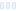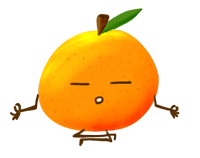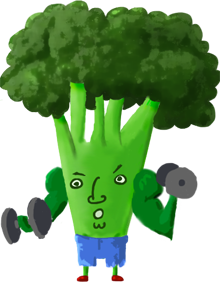Toddler Formula

with Iron, Original - Meijer

Main info:

Toddler Formula
with Iron, Original - Meijer
1 Serving
100 Calories
10.5 g
5.3 g
2.5 g

0 g
0 mg
0 g
36 mg
0 g
0 g

Percent calories from...
Nutrition Facts
For a Serving Size of (g)
How many calories are in Toddler Formula? Amount of calories in Toddler Formula: Calories Calories from Fat (%)
% Daily Value *
How much fat is in Toddler Formula? Amount of fat in Toddler Formula: Total Fat
How much sodium is in Toddler Formula? Amount of sodium in Toddler Formula: Sodium
How much potassium is in Toddler Formula? Amount of potassium in Toddler Formula: Potassium
How many carbs are in Toddler Formula? Amount of carbs in Toddler Formula: Carbohydrates
How many net carbs are in Toddler Formula? Amount of net carbs in Toddler Formula: Net carbs
How much fiber is in Toddler Formula? Amount of fiber in Toddler Formula: Fiber
How much protein is in Toddler Formula? Amount of protein in Toddler Formula: Protein
Vitamins and minerals
How much Vitamin A is in Toddler Formula? Amount of Vitamin A in Toddler Formula: Vitamin A
How much Vitamin C is in Toddler Formula? Amount of Vitamin C in Toddler Formula: Vitamin C
How much Calcium is in Toddler Formula? Amount of Calcium in Toddler Formula: Calcium
How much Iron is in Toddler Formula? Amount of Iron in Toddler Formula: Iron
Fatty acids
Amino acids
* The Percent Daily Values are based on a 2,000 calorie diet, so your values may change depending on your calorie needs.Loading similar foods...
Note: Any items purchased after clicking our Amazon buttons will give us a little referral bonus. If you do click them, thank you!Be cool

- Zen orangeI never skip arm day

- Buff broccoli